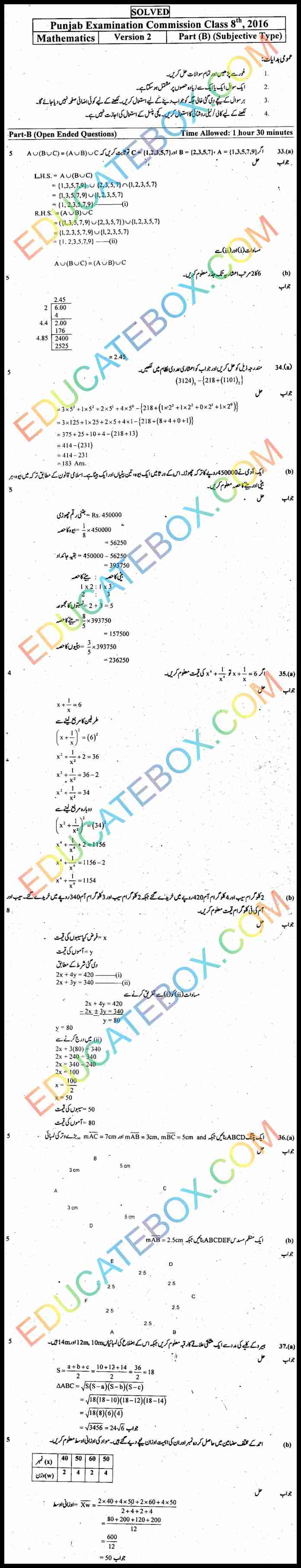# Past Paper 8th Class Maths 2016 Solved Paper UM Punjab Board (PEC) Version 2

0
1234

## Past Paper 8th Class Maths 2016 Solved Paper Punjab Board (PEC) Objective Type Urdu Medium Version 2

This paper was held on Wednesday, February 17, 2016 under the supervision of Punjab Examination Commission. Students can get help with this solved paper to understand different concepts. This is for those students whose medium is Urdu. This Urdu medium Maths paper has total hundred marks. Subjective part has 48 marks. Objective part has 52 marks.  Objective part has time duration of 1 hour and 15 minutes while for subjective part; the time duration is 1 hour and 30 minutes.

Some of the question in this paper include calculate subset of {3,4}, calculate power set of {0,4}, calculate rational number of a number, calculate square of 23, another square question of 12.25, calculate binary multiplication and division, binary addition, calculate octal conversion, and octal multiplication, Zaqat conversion, conversion of dollar and rupee, mode and median of data.

In the subjective part there are some simple Union questions. A square root question of  6 upto 2 decimal places. There is a conversion of a number to decimal number system question. Another question is a calculation of area of triangle. And there are few tricky statement questions.

## Past Paper 8th Class Maths 2016 Solved Paper Punjab Board (PEC) Subjective Type Urdu Medium Version 2 اپ ٹو ڈیٹ پیپر ہشتم کلاس ریاضی حل شدہ پیپر پنجاب بورڈ سبجیکٹیو ٹائپ اردو میڈیم ورژن 2